# RBSE Maths Class 8 Chapter 15: Important Questions and Solutions

RBSE Maths Chapter 15 – Surface area and Volume Mathematics Class 8 Important questions and solutions are available here. The additional, exercise important questions and solutions of Chapter 15, available at BYJU’S, contain detailed explanations. It can be used as a most useful reference for facing the board exams. All these important questions are based on the new pattern designed by the RBSE. Students can also get the syllabus and textbooks on RBSE Class 8 solutions.

Chapter 15 of the RBSE Class 8 Maths will help the students to solve problems related to the cuboids, the total surface area of a cube, surface area of a cylinder, volume of standard units, volume of cube and cuboid, the volume of a cylinder.

### RBSE Maths Chapter 15: Exercise 15.1 Textbook Important Questions and Solutions

Question 1: On the basis of the given measurements, determine the surface area of a cuboidal wooden log, cuboidal brick and box.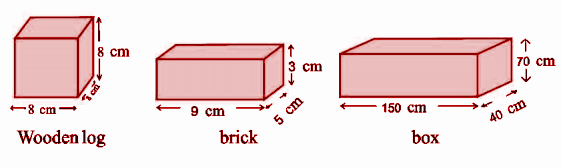Solution:

(i) Surface area of the cubical wooden log = 6 * (a)2

= 6 * (8)2

= 6 * 64

= 384 cm2

(ii) Surface area of cuboidal brick = 2 * (lb + bh + hl)

= 2 * (9 * 5 + 5 * 3 + 3 * 9)

= 2 * (45 + 15 + 27)

= 2 (87)

= 174 cm2

(iii) Surface area of cuboidal box = 2 * (lb + bh + hl)

= 2 * (150 * 40 + 40 * 70 + 70 * 150)

= 2 (6000 + 2800+ 10500)

= 2 (19300)

= 38600 cm2

Question 2: Determine the side of a cube whose total surface area is 600 square cm.

Solution:

Let a be the length of the side of the cube.

Total surface area of cube = 6a2 cm2

According to the question,

6a2 = 600

a2 = 600 / 6

a = √100

a = 10

Hence, the required length of the side of the cube is 10 cm.

Question 3: In the given figures below, whose surface area is the greatest?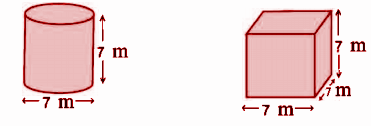Solution:

(i) In the first figure (cylinder),

r = [7 / 2] meters

r = 3.5 meters

h = 7 meter

Surface Area = 2πr (r + h)

= 2 * 3.14 * 3.5 (3.5 + 7)

= 21.98 [10.5]

= 230.79m2

(ii) In the second figure (cube),

a = 7 meters

Surface area = 6a2

= 6 * 2

= 6 * 49

= 294 m2

Hence, the surface area of the cube is greater than the surface area of the cylinder.

Question 4: Find the area of a curved surface if the area of the base of the cylindrical tank is 176 cm2 and height is 30 cm.

Solution:

Let r cm be the radius of the base.

The circumference of base = 2πr cm

According to the question,

2πr = 176

2 * 3.14 * r = 176

6.2831 * r = 176

r = 176 / 6.2831

r = 28.01 cm

Area of curved surface = 2πrh

= 2 * 3.14 * 28.01 * 30

= 5279.76 cm2

Question 5: From a sheet of 8 square meters, a closed cylindrical tank is formed which has one-meter height and 140 cm diameter. How many sheets will be left after making a tank?

Solution:

Diameter of cylindrical tank = 140 cm

Radius (R) =  /  = 70cm

Height = 1 meter = 100 cm

Surface area of cylindrical tank = 2πr (r + h)

= 2 * 3.14 * 70 * [70 + 100]

= 74769 cm2

= 7.48 m2

The surface area of the metal sheet = 8 m2

Surface area of remaining sheet = 8 – 7.48 = 0.52 m2

Question 6: How many paint tins having the spread capacity of 100cm2 will be required to paint the external surface of the box with dimensions 80 cm * 50 cm * 25 cm?

Solution:

Area of outer surface of box = 2 * (lb +bh + lh)

= 2 * (80 * 50 + 50 * 25 + 25 * 80)

= 2 * (4000+ 1250 + 2000)

= 2 * (7250)

= 14500 cm,

Spread capacity of 1 paint box = 100 cm2

Required number of paint boxes = [Area of outer surface of the box] / [capacity of 1 paint box]

= 14500 / 100

= 145 paint tins will be required to paint the external surface of the box.

Question 7: There are 25 cylindrical pillars in a building. Each pillar has a radius of 28 cm and a height of 4 m. Find the expenditure of painting curved surface area of all the pillars at the rate of Rs. 8 per meter square.

Solution:

For one cylindrical pillar, radius (r) = 28 cm

Height (h) = 4 m = 4 * 100 cm = 400 cm

Curved surface area of 1 pillar = 2πrh

= 2 * π * 28 * 400

= 70400 cm2

Curved surface area of 25 cylindrical pillars = 70400 x 25

= 1760000 cm2

= 176 m2

Cost of painting of 25 cylindrical pillars = 176 * 8 = Rs. 1408.

Question 8: Curved surface area of a hollow cylinder is 4224 cm2. A rectangular sheet having width 33 cm is formed, cutting it along its height. Find the perimeter of the sheet.

Solution:

Let r meter be the radius of the base of cylinder and height be h meter.

The surface area of cylinder = 2πrh cm2

According to the question,

2πrh = 4224

2 * π * r * (33) = 4224

2πr = 4224 / 33

2πr = 128

Perimeter of base = 128 cm

Length of sheet (l) = 128 cm

Breadth of sheet (b) = 33 cm

Perimeter of rectangular sheet = 2 * (I + b)

= 2 * (128 + 33)

= 2 * (161)

= 322 cm

Question 9: To make a road plain, a roller has to complete 750 rounds. If the diameter of the roller is 84 cm and length 1 meter, then find the area of the road.

Solution:

Diameter of roller = 84 cm

Radius (r) = d / 2 cm = 84 / 2 = 42 cm

Length (h), 1 meter = 100 cm

Curved surface area = 2πrh

= 2 * π * 42 * 100

= 26400 cm2

Area of road that is made plane in one round = 26400 cm2.

Area of road that is made plane in 750 rounds = 26400 x 750 = 19800000 cm2 = 1980m2.

Question 10: A cube is made by arranging 64 cubes having a side of 1 cm, find the total surface area of the cube so formed.

Solution:

Side of one cube = 1 cm

Volume of one cube = a3 = (1)3 = 1 cm3

Volume of 64 cubes = 64 * 1 = 64 cm3

Let the side of the new cube be x cm.

Volume of new cube = Volume of 64 cubes

=>x3 = 64 cm

x = (64)1/3

x = 4cm

Total surface area of new cube = 6a2

= 6 * (4)2

= 6 * 16

= 96 cm2

### RBSE Maths Chapter 15: Exercise 15.2 Textbook Important Questions and Solutions

Question 1: The dimensions of a cuboid are 60 cm * 54cm * 30 cm. How many cubes of side 6 cm can be placed in the cuboid?

Solution:

Volume of the cuboid = 60 cm * 54cm * 30 cm = 97200 cm3

Volume of the cube of side 6 cm = (6)3 = 216 cm3

The required number of cubes = Volume of cuboid / Volume of one cube

= 97200 / 216

= 450 cubes

Question 2: How many wooden logs of side 6 cm can be cut from a 3 m long, 50 cm broad and 25 cm high wooden pile.

Solution:

Volume of wooden pile = 300 * 50 * 25 cm [1 meter = 100 cm]

= 375000 cm

Volume of 1 cubical block = 25 * 25 * 25 = 15625 cm3

Required number of cubical blocks = [Volume of wooden pile / Volume of 1 block]

= [375000 / 15625]

= 24

Question 3: Cylinder A has a diameter of 14 cm end height of 7 cm. Cylinder B has a diameter 7 cm and height 14 cm. Without any calculation, determine the volume of the cylinder that is the greatest? Verify the answer by calculation.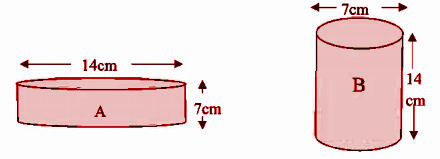Solution:

For cylinder A, the radius of base (r), = [14 / 2] cm = 7 cm

Height (h) = 7 cm

Volume of the cylinder = πr2h

= [22 / 7] * (7)2 * (7)

= 1078 cm3

For cylinder B, the radius of base r = [7 / 2] cm

Height (h) = 14 cm

Volume of the cylinder = πr2h

= [22 / 7] * [7 / 2]2 * 14

= [22 / 7] * [49 / 4] * 14

= 539 cm3

Hence, the volume of cylinder A is 1078 cm3 and the volume of cylinder B is 539cm3.

So, the volume of cylinder A > the volume of cylinder B.

Question 4: From a cylindrical milk tanker of radius 1.5 m and length 7 m, how many polythenes of one litre can be packed, (1m3 = 1000 litre)

Solution:

Radius of the tanker = 1.5 meter

Height (h) = 7 meter

Volume of the cylinder = πr2h

= [22 / 7] * (1.5) * (1.5) * 7

= 49.5 m3

= 49.5 x 1000 litre

= 49500 litre

Hence, the required number of polythene packs are 49500.

Question 5. In what time can a tap giving 60 litres of water per minute fill a cylindrical tank of radius 3.5 m and depth 3 m?

Solution:

Radius of the tank = 3.5 meter

Depth of the tank (h) = 3 meter

Volume of the cylinder = πr2h

= [22 / 7] * (3.5) * (3.5) * 3

= 115.5 m3

= 115.5 x 1000 litre

= 115500 litre

Required time = [Volume of the tank] / [Volume of water flowing in a minute]

=  / 

= 1925 minutes

Question 6: Dimensions of a cuboidal ice is 50 cm * 30 cm * 20 cm. Find its weight in kilograms if the weight of 1000 cm3 ice is 900 gram.

Solution:

Volume of the ice plate = 50 * 30 * 20

= 30000 cm3

Weight of the ice plate = 30000 * [900 / 1000]

= 27000 grams

= 27000 / 1000 [1kg = 1000 grams]

= 27 kg

Question 7: If the side of a cube is doubled then,

(i) How many times will its surface area increase?

(ii) How many times will its volume increase?

Solution:

(i) Let a cm be the side of the cube.

Surface area S1 = 6a2 cm2

New side = 2a cm

New surface area S2 = 6 * (2a)2 = 24 a2 cm2

S2 / S1 = 24a2 / 6a2 = 4

S2 = 4S1

So, the surface area of the new cube will increase by 4 times.

(ii) Volume V1 = a3 cm3

New volume = V2 = (2a)3 cm3

= 8a3 cm3

V2 / V1 = 8a3 / a3 = 8

V2 = 8V1

So, the volume increases by 8 times.

Question 8: Volume of a cylindrical tank having 7-meter diameter is 770 cubic meters. Find the height of the tank.

Solution:

The diameter of the tank [d] = 7 meters

The radius of the tank = d / 2 = 7 / 2 m = 3.5 m

Let the height of the tank be ‘h’ m.

The volume of the cylindrical tank = πr2h

770 = [22 / 7] * (3.5) * (3.5) * h

770 = 38.5 * h

770 / 38.5 = h

h = 20 m

The height of the tank is 20 meters.

### RBSE Maths Chapter 15: Additional Questions and Solutions

Question 1: If the cuboid is formed by sticking 3 sides of a cube ‘x’, then what will be the dimensions of the cuboid?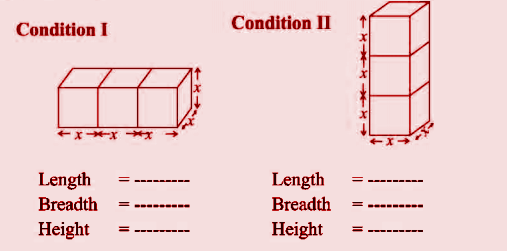Solution:

Condition I

Length = 3x

Height = x

Condition II

Length = x

Height = 3x

Question 2: Find the surface area of a cube having side 3 cm. What will be the surface area of 5 such cubes?

Solution:

Surface area of cube of side 3 cm = 6a2

= 6 * (3)2

= 6 * 9

= 54 cm

Surface area of 5 cubes = 54 * 5 = 270 cm2

Question 3: What will be the total surface area of 5 cubes having side 3 cm.? If they are stocked one after one, then how much surface area will be decreased?

Solution:

Surface area of cube of side 3 cm = 6a2

= 6 * (3)2

= 6 * 9

= 54 cm

Surface area of 5 cubes = 54 * 5 = 270 cm2

If 5 cubes are joined one after one, then it becomes a cuboid of length [l]

= 3 cm + 3 cm + 3 cm + 3 cm + 3 cm

= 15cm

Height (h) = cm

Surface area = 2 * (lb + bh + lh)

= 2 * (15 * 3 + 3 * 3 + 3 * 15)

= 2 * (45 + 9 + 45)

= 198 cm2

Hence, the surface area will be reduced and the difference = 270 cm2 – 198 = 72 cm2.

Question 4: Find the lateral surface area of the cuboid given below.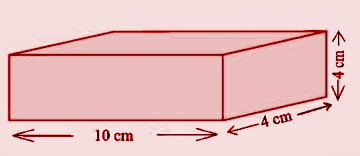Solution:

Length of the cuboid = 10 cm.

Breadth of the cuboid = 4cm

Height of the cuboid = 4 cm

The lateral surface area of cuboid = 2 * (l + b) *h

= 2 * (10 + 4) * (4)

= 2 * 14 * 4

= 112 cm2

Question 5: Determine the total surface area of the following cylinders.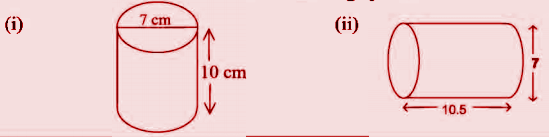Solution:

[i] In the figure [i], the radius of the cylinder = diameter / 2 = 7 / 2 = 3.5 cm

Height of cylinder [h] = 10cm

Total surface area of the cylinder = 2πr (r + h)

= 2 * [22 / 7] * [3.5] * [3.5 + 10]

= 297 cm2

[ii] In the figure [ii], the radius of the cylinder = diameter / 2 = 7 / 2 = 3.5 cm

Height of cylinder [h] = 10.5 cm

Total surface area of the cylinder = 2πr (r + h)

= 2 * [22 / 7] * [3.5] * [3.5 + 10.5]

= 308 cm2

Question 6: By arranging the cubes of unit length, find the volume of the cubes so obtained.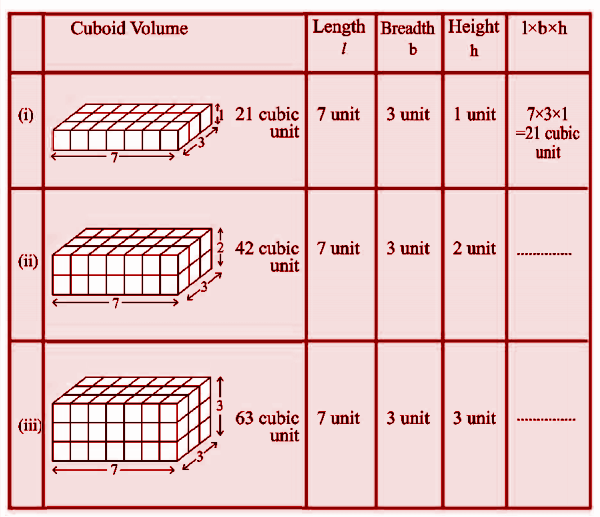Solution:

 Sl.no Cuboid Volume Length [L] Breadth [B] Height [H] L * B * H [cubic unit] [i] 21 cubic unit 7 units 3 units 1 unit 7 * 3 * 1 = 21 [ii] 42 cubic unit 7 units 3 units 2 units 7 * 3 * 2 = 42 [iii] 63 cubic unit 7 units 3 units 3 units 7 * 3 * 3 = 63

Question 7: Find the volume of the given cuboids on the basis of the given measures.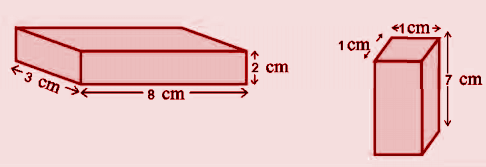Solution:

(i) Length of the cuboid [L] = 8 cm

Breadth of the cuboid [B] = 3 cm

Height of the cuboid [H] = 2 cm

Volume of the cuboid = L * B * H = 8 * 3 * 2 = 48 cm3

(ii) Length of the cuboid [L] = 1 cm

Breadth of the cuboid [B] = 1 cm

Height of the cuboid [H] = 7 cm

Volume of the cuboid = L * B * H = 1 * 1 * 7 = 7 cm3

Question 8: Complete the following table.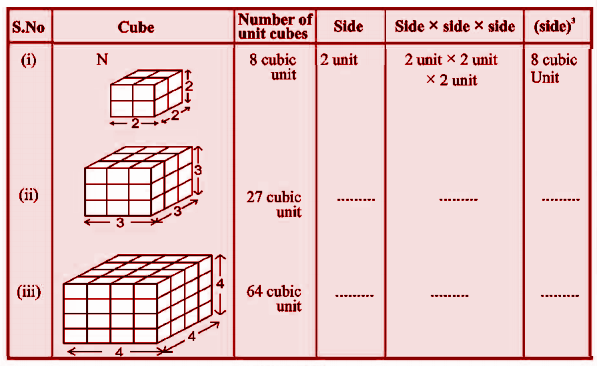Solution:

 Sl.no Cube Number of unit cubes Side Side * Side * Side (Side)3 [i]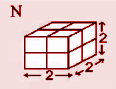8 cubic units 2 unit 2 * 2 * 2 8 cubic units [ii]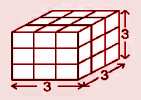27 cubic units 3 3 * 3 * 3 27 cubic units [iii]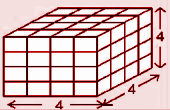64 cubic units 4 4 * 4 * 4 64 cubic units

Question 9: Determine the volume of the cube having side.

[i] 1.5cm

[ii] 4m

Solution:

(i) 1.5 cm

Here, a = 1.5 cm

Volume of cube = a3

= (1.5)3

= 1.5 * 1.5 * 1.5

= 3.375 cm3

(ii) 4 cm

Here a = 4 cm

Volume of cube = a3

= (4)3

= 4 * 4 * 4

= 64 cm3

Question 10: Match the following.

 Question Answer Choices 1] Number of vertices of a cube A] 6m 2] Number of edges of a cube B] 2 3] Number of edges of the cylinder C] 12 4] Side of a cube of volume 216 m3 D] 8

Solution:

1] – D]

2] – C]

3] – B]

4] – A]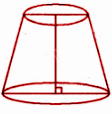Solution:

A cylinder consists of two edges and circles which are congruent. In the above figure, the circles are not congruent. Hence, it is not a cylinder.

Question 12: The ratio of two cylindrical poles is 3:2, and the ratio of their heights is 2:3. Find the ratio of the curved surface area of poles.

Solution:

S1 / S2 = 2πr1h1 / 2πr2h2

= [r1 / r2] * [h1 / h2]

= [3 / 2] * [2 / 3]

= 1:1

Question 13: The area of three adjacent faces of a cuboid are p, q and r. The volume of the cuboid is V. Prove that V2 = pqr.

Solution:

Let Iength [l], breadth [b] and height [h] be the dimensions of the cuboid respectively.

Then, p = lb, q = bh, r = lh

p* q * r = (Ib) (bh) (lh)

= l2b2h2

= V2

So, V2 = pqr

Question 14: If V is the volume of a cuboid of dimensions a, b and c, surface area is S, then prove that [1 / V] = [2 / s] * [(1 / a) + (1 / b) + (1 / c)].

Solution:

LHS = [1 / V] = [1 / abc] —– (1)

RHS = [2 / s] * [(1 / a) + (1 / b) + (1 / c)]

= [2 / 2 [ab + bc + ca]] * [(bc + ca + ab) / abc)]

= 1 / abc —– (2)

From (1) and (2), LHS = RHS

[1 / V] = [2 / s] * [(1 / a) + (1 / b) + (1 / c)]

Question 15: The population of a village is 2000. Every citizen needs 150 litres of water per day. The dimensions of the water tank are 20 m * 15 m * 6 m in the village. For how many days is this tank sufficient for the village?

Solution:

Volume of the tank = 20 m * 15 m * 6 m

= 1800 m3

= 1800 * 1000 litres

Water requirement of village per day = 150 * 2000 = 300,000 litres

Required number of days = Volume of the tank / Requirement of people per day

= [1800 * 1000] / 

= 6 days

Question 16: A closed iron tank of dimensions 12 m in length, 9 m in breadth and 4 m in depth is to be made. The iron sheet whose cost is Rs 50 per meter and which is 2 m wide is used. Find the total cost of the iron sheet required for the iron tank.

Solution:

Surface area of tank = 2 * (lb + bh + lh)

= 2 * (12 * 9 + 9 * 4 + 4 * 12)

= 2 * (108 + 36 + 48) = 2 (192)

= 384 m3

Width of iron sheet = 2 meter

Length of iron sheet = 384 / 2 = 192 meter

Cost of iron sheet = 192 * 50 = Rs 9600.

Question 17: A cylinder, whose height is 3 m, is open from the top. The circumference of its base is 22 m. Find the total surface area.

Solution:

Let the radius of the base be ‘r’ m.

2𝛑r = 22

 * [22 / 7] x r = 22

6.286 * r = 22

r = 3.5 cm

Total Surface Area = 2𝛑rh + 𝛑r2

= 2 * [22 / 7] * [3.5] * 3 + [22 / 7] * [3.5]2

= 66 + 38.5

= 104.5 m2

Question 18: A cylindrical pipe, which is open from both sides is made of an iron sheet of thickness 2 cm. If the external diameter is 16 cm and height is 100 cm, then find the total iron used in making the pipe.

Solution:

External diameter = 16 cm.

External radius (R) = [d / 2] = [16 / 2] cm = 8 cm

Thickness of the sheet = 2 cm

Inner radius (r) = 8 – 2 = 6 cm

Height (h) = 100 cm

Used iron = Extemal volume – Internal Volume

= 𝛑R2h – 𝛑r2h

= 𝛑 * [R2 – r2] * h

= [22 / 7] * [82 – 62] * 100

= [22 / 7] * [64 – 36] * 100

= 8800 cm3

Question 19: Find the length of the longest rod, which can be put in a room of dimensions 12 m * 9 m * 8 m.

Solution:

The given room is of

Length = 12m

Height = 8m

Length of the longest rod = Length of the diagonal = √l2 + b2 + h2

= √122 + 92 + 82

= √144 + 81 + 64

= √289

= 17 m

Question 20: The curved surface area of a cylindrical tank is 440m2. Its height is 4 m. Find the volume of the task.

Solution:

Height of cylinder (h) = 4 m

Curved surface area of cylinder = 440 m2

Volume of the cylinder has to be found.

2𝛑rh = 440

2 * [22 / 7] * r * 4 = 440

25.143 * r = 440

r = 440 / 25.143

r = 17.5 m

Volume of the cylinder = 𝛑r2h

= [22 / 7] * [17.5]2 * 4

= 962.5 * 4

= 3850 m2

Question 21: Determine the side of a cube whose total surface area is 1014 cm2. Find its volume also.

Solution:

Let the side of the cube be ‘a’ cm.

The total surface area of cube = 6a2

According to question,

6a2 = 1014

a2 = 1014 / 6

a2 = 169

a = 13 cm

Volume of the cube = (side)3 = [13 * 13 * 13]3 = 2197 cm3

Question 22: Radius and total surface area of a cylinder are 7 cm and 968 cm2 respectively. Find its height.

Solution:

Given, radius of the cylinder (r) = 7 cm

Total surface area = 968 cm2

Height (h) = ?

Total surface area of cylinder = 2πr (r + h)

968 = 2 * [22 / 7] * 7 (h + 7)

968 = 6.286 * [7h + 49]

968 – 308.014 = 44.002 * h

659.986 / 44.002 = h

h = 15cm

Height of cylinder = 15 cm.

Question 23: Curved surface area of a cylinder is 880 m2 whose height is 10 m. Find the volume of a cylinder.

Solution:

The curved surface area of cylinder = 880 m2 and height (h) = 10 meters.

2πrh = 880 m

2 * [22 / 7] * r * 10 = 880

62.86 * r = 880

r = 880 / 62.86

r = 14

Volume of the cylinder = πr2h

= [22 / 7] * 2 * 10

= 6160 m3